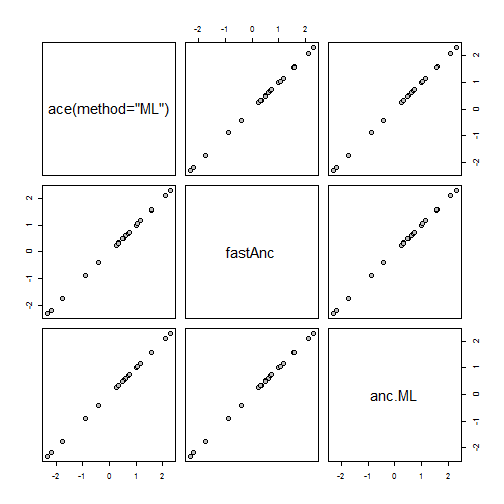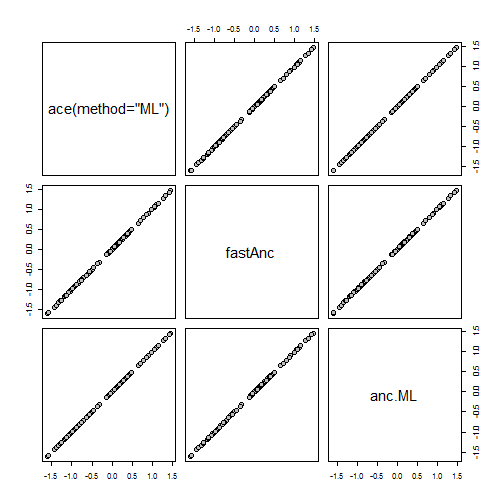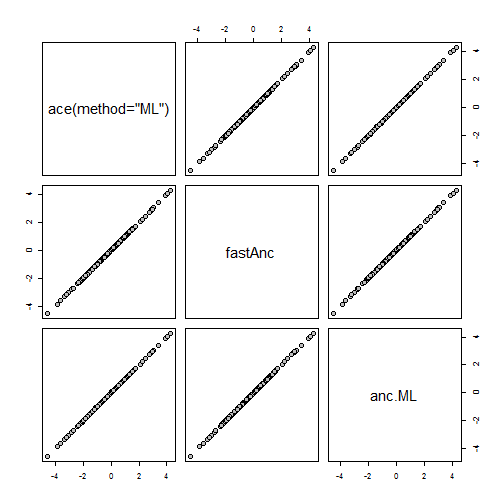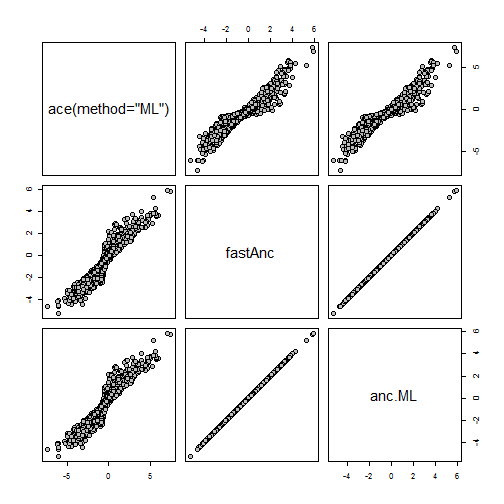## Sunday, June 11, 2017

### ML estimation of ancestral states for large trees using `ace`

A phytools user recently contacted me to report differences between several different functions in R to estimate ancestral states at internal nodes for continuous characters using likelihood.

Since I had no idea to expect this, I ran a quick comparison of `ace(method="ML")`, `fastAnc`, and `anc.ML`. Ostensibly, all three methods are performing ML estimation assuming a Brownian model of evolutionary change. The only difference is the method of optimization. `fastAnc`, for example, using a 're-rooting method' in which the tree is re-rooted at every internal node and the contrasts algorithm is used to obtain the ML state for that node. `anc.ML`, also in phytools, uses numerical optimization - but initiates the search using the values from `fastAnc`.

It occurred to me immediately that the problem may be related not to the implementation of the model, but rather to the simple issue of numerical optimization. Here is a demo suggesting that this could indeed be the case:

``````library(phytools)

## tiny example:
tree<-pbtree(n=26,tip.label=LETTERS)
x<-fastBM(tree)
fit1<-ace(x,tree,type="continuous",method="ML")
fit2<-fastAnc(tree,x,vars=TRUE,CI=TRUE)
fit3<-anc.ML(tree,x)
obj<-cbind(fit1\$ace,fit2\$ace,fit3\$ace)
colnames(obj)<-c("ace(method=\"ML\")","fastAnc","anc.ML")
pairs(obj,pch=21,bg="grey",cex=1.5)
````````````## small example
tree<-pbtree(n=100)
x<-fastBM(tree)
fit1<-ace(x,tree,type="continuous",method="ML")
fit2<-fastAnc(tree,x,vars=TRUE,CI=TRUE)
fit3<-anc.ML(tree,x)
obj<-cbind(fit1\$ace,fit2\$ace,fit3\$ace)
colnames(obj)<-c("ace(method=\"ML\")","fastAnc","anc.ML")
pairs(obj,pch=21,bg="grey",cex=1.5)
````````````## medium example
tree<-pbtree(n=200)
x<-fastBM(tree)
fit1<-ace(x,tree,type="continuous",method="ML")
fit2<-fastAnc(tree,x,vars=TRUE,CI=TRUE)
fit3<-anc.ML(tree,x)
obj<-cbind(fit1\$ace,fit2\$ace,fit3\$ace)
colnames(obj)<-c("ace(method=\"ML\")","fastAnc","anc.ML")
pairs(obj,pch=21,bg="grey",cex=1.5)
````````````## large example:
tree<-pbtree(n=1000)
x<-fastBM(tree)
fit1<-ace(x,tree,type="continuous",method="ML")
``````
``````## Warning in sqrt(diag(solve(h))): NaNs produced
``````
``````fit2<-fastAnc(tree,x,vars=TRUE,CI=TRUE)
fit3<-anc.ML(tree,x)
obj<-cbind(fit1\$ace,fit2\$ace,fit3\$ace)
colnames(obj)<-c("ace(method=\"ML\")","fastAnc","anc.ML")
pairs(obj,pch=21,bg="grey",cex=1.5)
``````What we should see is that the results are identical for 'tiny' and relatively modest-sized trees, but go awry for large & possibly medium-sized trees where the number of parameters (the states at all the nodes) really explodes. This might suggest that the problem is with numerical optimization on large trees, rather than an issue with how the model is implemented or how the likelihood computed.

This should serve as a reminder that any method depending on numerical optimization is only as good as its optimization routine.